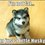How many solutions are there to $Z^{n-1} =i \overline{Z}$ and what are the solutions?Note by Anik Mandal
5 years, 4 months ago

This discussion board is a place to discuss our Daily Challenges and the math and science related to those challenges. Explanations are more than just a solution — they should explain the steps and thinking strategies that you used to obtain the solution. Comments should further the discussion of math and science.

When posting on Brilliant:

• Use the emojis to react to an explanation, whether you're congratulating a job well done , or just really confused .
• Ask specific questions about the challenge or the steps in somebody's explanation. Well-posed questions can add a lot to the discussion, but posting "I don't understand!" doesn't help anyone.
• Try to contribute something new to the discussion, whether it is an extension, generalization or other idea related to the challenge.

MarkdownAppears as
*italics* or _italics_ italics
**bold** or __bold__ bold
- bulleted- list
• bulleted
• list
1. numbered2. list
1. numbered
2. list
Note: you must add a full line of space before and after lists for them to show up correctly
paragraph 1paragraph 2

paragraph 1

paragraph 2

[example link](https://brilliant.org)example link
> This is a quote
This is a quote
    # I indented these lines
# 4 spaces, and now they show
# up as a code block.

print "hello world"
# I indented these lines
# 4 spaces, and now they show
# up as a code block.

print "hello world"
MathAppears as
Remember to wrap math in $$ ... $$ or $ ... $ to ensure proper formatting.
2 \times 3 $2 \times 3$
2^{34} $2^{34}$
a_{i-1} $a_{i-1}$
\frac{2}{3} $\frac{2}{3}$
\sqrt{2} $\sqrt{2}$
\sum_{i=1}^3 $\sum_{i=1}^3$
\sin \theta $\sin \theta$
\boxed{123} $\boxed{123}$

Sort by:

Multiply both sides by $z$ to get $z^n = i|z|^2 \Rightarrow |z| = 1$ after considering the modulus of both sides. So, we get $z^n = i$ which are just the nth roots of $i$.

- 5 years, 4 months ago

How to find the nth roots of $i$?

- 5 years, 4 months ago

Sorry, I should have added that. Express $i$ as $e^{i\pi/2}$, and let $z = e^{i\theta}$ We have n roots given by,
( $k$ goes from $0$ to $n - 1$):
$z^n = e^{i\pi/2} \\ \Rightarrow e^{in\theta} = e^{i\pi/2} \\ \Rightarrow n\theta = 2k\pi + \pi/2 \\ \Rightarrow \theta = \frac{(4k+ 1)\pi}{2n} \\ \Rightarrow z_k = e^{\frac{i(4k+ 1)\pi}{2n}}$

- 5 years, 4 months ago

I am not too familiar with complex numbers..Can you please explain why $n\theta = 2k\pi+\frac{\pi}{2}$

- 5 years, 4 months ago

Sure!
When you look at the complex plane, you see this:the complex plane Each point in this plane determines a unique complex number.
For a point, the x coordinate determines the real part, and the y coordinate determines the imaginary part. In the figure, we have $z = x + iy$.
This is called the Cartesian representation of a complex number. We can also represent complex numbers in another form, called the polar form.
In the polar form, each number is represented by two parameters, its modulus (r in the figure, the distance from the origin) and its argument ($\phi$, the angle shown in the figure). By varying these parameters, we can reach every complex number.
But if you think about it, how can the argument take a unique value for a specific $z$?
Indeed, if we rotated the angle by $2\pi$ radians then we would get the same number again. If we rotated it by $2\pi$ again, for a total of $4 \pi$ radians, we would get $z$ again! So we've seen that the argument is not affected by adding a multiple of $2\pi$.
Keep this in mind whenever we think about arguments of complex numbers.

We need to find a way to relate the two representations. Trigonometry is the way!
Looking at the right-angled triangle in the figure,
$x = r \cos \phi$, and $y = r \sin \phi$, so, $z = r( \cos \phi + i\ sin \phi )$
It looks neat.
But where does $e^{i\phi}$ come from? The answer is here, Euler's formula.
This is the reason why complex numbers are so powerful. With this, we have,
$e^{i\phi} = (\cos \phi + i\ sin \phi )$, so $z = r e^{i\phi}$
If I'm saying $z_1 = r_1e^{i\phi_1} = z_2 = r_2e^{i\phi_2}$, I mean their moduli are equal, but their arguments could differ by a multiple of $2\pi$. So $\phi_1 - \phi_2 = 2k\pi$ for some integer $k$.

This is where $n\theta = 2k\pi + \frac{\pi}{2}$ comes from, $n \theta$ is the same angle as $\frac{\pi}{2}$ upto a multiple of $2\pi$.

- 5 years, 4 months ago

That's was really helpful Sir!Thanks a lot! Sir one last doubt : Why was it k from 0 to $n-1$?

- 5 years, 4 months ago

A polynomial of degree n has only n zeroes.

Thus k is from 0 to (n-1).

- 5 years, 4 months ago

Why not 1 to n?

- 5 years, 4 months ago

$k = 0$ and $k = n$ give the same root (arguments differ by $2\pi$).

- 5 years, 4 months ago

$e^{ix} = cosx +isinx$

Put $x=pi/2,5pi/2,9pi/2 \cdots ,2kpi +pi/2$, all will yeild i.

Hope this helps.

- 5 years, 4 months ago

Yes i have understood!Thanks for answering..

- 5 years, 4 months ago

- 5 years, 4 months ago

Smooth observation, missed that during the multiplication. Then we also require $n > 1$, though.

- 5 years, 4 months ago

Yeah, thanks for pointing that out.

- 5 years, 4 months ago